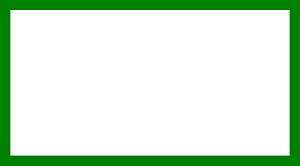# Area of a Rectangle

Instructions: Use this calculator to find the area of a rectangle, with a given sides a and b. Please provide the sides in the boxes below.One side $$a$$ of the rectangle (Ex: 2, or 3/2, etc.)The other side $$b$$ of the rectangle (Ex: 2, or 3/2, etc.)

This calculator will compute the area of a rectangle, for the two sides a and b that you provide. The provided sides of the rectangle must be valid numeric expressions. For example you can use a number with or without decimals like '3' or '6.56', or you could use algebraic expressions like '1/3', or 'sqrt(3)', etc.

Once you have provided two valid numbers or expressions, you just have to click on the "Calculate" button, and all the steps of the calculation will be shown.

Knowing how to compute the area of a rectangle and the area of a square are among the most crucial core abilities that we learn in Algebra, and it is for a good reason.## How do compute the area of a rectangle?

The actual calculation process is quite simple, and it just involves multiplying the two sides together. So, if the sides of the rectangles are $$a$$ and $$b$$, then the formula for the area of a rectangle is

$\text{Area} = a b$

## What are the steps for computing the area of a rectangle

• Step 1: Identify the sides of the rectangle that are being provided, and call them 'a' and 'b'
• Step 2: Once you know the sides 'a' and 'b', the area is computed a * b
• Step 3: If needed, identify the units of 'a' and 'b' (if any) and give units to the area

## What would be the purpose of the calculation of the area of a rectangle?

Calculus and Algebra provide numerous examples of where the calculation of areas of rectangles and squares is vital. You may be simply computing areas, or you may be solving an optimization problem (like finding the maximum area that can be enclosed with a given amount of fencing, just to given one example).

Also, the calculation of the areas of rectangles gives the foundation for the processes of Calculus.### Example: Calculate the area of a rectangle

Calculate the area of a rectangle with given sides a = 4 and b = 5.

Solution: We need to first identify the sides of the rectangle. In this case, it is clearly specified that the sides are a = 5 and b = 5. Then, the appropriate formula for the calculation of the area is:

$Area = a b$

Then, we plug in the values a = 4 and b = 5 into the formula:

$Area = a b = 4 \times 5 = 20$

so the area of the rectangle is 20.

### Example: Area of a rectangle with units

Calculate the area of a rectangle with sides of a = 4.2 cm and b = 6 cm

Solution: As usual, we first identify the sides of the rectangle, which in this case are a = 4.2 cm and b = 6 cm. So then in this case, the sides come with length units (cm). The formula is the same one:

$Area = a b$

Then, by plugging a = 4.2 cm and b = 6 cm into the formula, we get that:

$Area = a b = 4.2 \times 6 \,\, cm^2 = 25.2 \,\,cm^2$

which indicates that the area is 25.2 cm2.

## Other practical area calculators

You will likely find the need of compute the areas of other basic geometric areas. For example you may be interested in finding the area of a rhombus and the area of a triangle, which use a very similar strategy as the one used by squares and rectangles.

For shapes that don't have straight borderlines, the strategy is different, and the constant $$\pi$$ plays a role. Indeed, you can use our area of circle calculator as well as the area of an ellipse calculator.